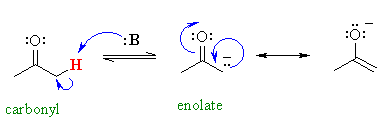# Find most acidic hydrogen?

##Feb 6, 2018

Identify familiar functional groups.

• $p$ is an amide proton. ${\text{pK}}_{a} \approx 15$
• $Y$ is an alkyl proton para to a carbonyl. ${\text{pK}}_{a} > 50$
• $X$ is an alkyl proton adjacent to a carbonyl. ${\text{pK}}_{a} \approx 20$
• $Z$ is an amine proton. ${\text{pK}}_{a} > 30$

Therefore, $p$ should be the most acidic. Its conjugate base is the weakest base here, and is thus the most stabilized.

http://cactus.dixie.edu/smblack/chem2310/summary_pages/pka_chart.pdf

Feb 6, 2018

The most acidic hydrogens are (d) p.

#### Explanation:

(b) Y

Protons Y are alkane hydrogens. They are the least acidic.

"p"K_text(a) ≈ 50

(c) Z

Protons Z are amine hydrogens.

They are slightly more acidic than alkanes because $\text{N}$ is more electronegative than $\text{C}$ and an $\text{N-H}$ bond is weaker than a $\text{C-H}$ bond.

"p"K_text(a) ≈ 35

(a) X

Protons X are alpha to a carbonyl group.

They are more acidic because the electrons of the conjugate base can be delocalized onto the adjacent carbonyl group to form a resonance-stabilized enolate ion.$\text{p} {K}_{\textrm{a}}$ values vary widely but for cyclohexanone "p"K_text(a) ≈ 17.

(d) p

Protons p are amide hydrogens.

The lone pair electrons can be delocalized onto the adjacent carbonyl group to form a resonance-stabilized anion.

"p"K_text(a) ≈ 15

Thus, protons p are the most acidic protons.